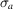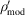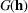International Tables for Crystallography (2006). Vol. F, ch. 15.1, pp. 311-324   | 1 | 2 | https://doi.org/10.1107/97809553602060000687

Contents

• 15.1. Phase improvement by iterative density modification  (pp. 311-324)
• 15.1.1. Introduction  (p. 311) | html | pdf |
• 15.1.2. Density-modification methods  (pp. 311-318) | html | pdf |
• 15.1.2.1. Solvent flattening  (pp. 311-314) | html | pdf |
• 15.1.2.1.1. Introduction  (pp. 311-313) | html | pdf |
• 15.1.2.1.2. The automated convolution method for molecular-boundary identification  (p. 313) | html | pdf |
• 15.1.2.1.3. The solvent-flattening procedure  (p. 314) | html | pdf |
• 15.1.2.2. Histogram matching  (pp. 314-316) | html | pdf |
• 15.1.2.2.1. Introduction  (p. 314) | html | pdf |
• 15.1.2.2.2. The prediction of the ideal histogram  (pp. 314-315) | html | pdf |
• 15.1.2.2.3. The process of histogram matching  (pp. 315-316) | html | pdf |
• 15.1.2.2.4. Scaling the observed structure-factor amplitudes according to the ideal density histogram  (p. 316) | html | pdf |
• 15.1.2.3. Averaging  (pp. 316-317) | html | pdf |
• 15.1.2.3.1. Introduction  (p. 316) | html | pdf |
• 15.1.2.3.2. The determination of noncrystallographic symmetry  (pp. 316-317) | html | pdf |
• 15.1.2.3.3. The refinement of noncrystallographic symmetry  (p. 317) | html | pdf |
• 15.1.2.3.4. The averaging of NCS-related molecules  (p. 317) | html | pdf |
• 15.1.2.4. Skeletonization  (pp. 317-318) | html | pdf |
• 15.1.2.5. Sayre's equation  (p. 318) | html | pdf |
• 15.1.2.5.1. Sayre's equation in real and reciprocal space  (p. 318) | html | pdf |
• 15.1.2.5.2. The application of Sayre's equation to macromolecules at non-atomic resolution – the θ() curve  (p. 318) | html | pdf |
• 15.1.2.6. Atomization  (p. 318) | html | pdf |
• 15.1.3. Reciprocal-space interpretation of density modification  (p. 319) | html | pdf |
• 15.1.4. Phase combination  (pp. 319-321) | html | pdf |
• 15.1.4.1. Sim andweighting  (p. 320) | html | pdf |
• 15.1.4.2. Reflection omit  (p. 320) | html | pdf |
• 15.1.4.3. The γ correction and solvent flipping  (pp. 320-321) | html | pdf |
• 15.1.5. Combining constraints for phase improvement  (pp. 321-323) | html | pdf |
• 15.1.5.1. The system of nonlinear constraint equations  (p. 321) | html | pdf |
• 15.1.5.2. Least-squares solution to the system of nonlinear constraint equations  (pp. 321-323) | html | pdf |
• 15.1.5.2.1. The conjugate-gradient method  (p. 322) | html | pdf |
• 15.1.5.2.2. The full-matrix solution  (pp. 322-323) | html | pdf |
• 15.1.5.2.3. The diagonal approximation  (p. 323) | html | pdf |
• 15.1.6. Example  (pp. 323-324) | html | pdf |
• References | html | pdf |
• Figures
• Fig. 15.1.2.1. Density-modification calculation showing iterative application of real-space and reciprocal-space constraints  (p. 311) | html | pdf |
• Fig. 15.1.2.2. Solvent mask determined from a map by Wang's method  (p. 313) | html | pdf |
• Fig. 15.1.2.3. Transformation of density ρ toby histogram matching  (p. 315) | html | pdf |
• Fig. 15.1.2.4. Types of noncrystallographic symmetry and averaging calculation  (p. 316) | html | pdf |
• Fig. 15.1.3.1. The functionsandfor solvent flattening, histogram matching and averaging  (p. 319) | html | pdf |
• Fig. 15.1.6.1. Phase correlations after different combinations of density modifications  (p. 323) | html | pdf |
• Tables
• Table 15.1.2.1. Constraints used in density modification  (p. 312) | html | pdf |